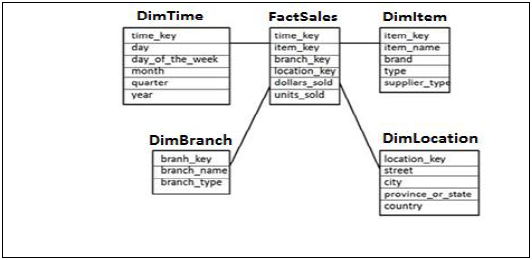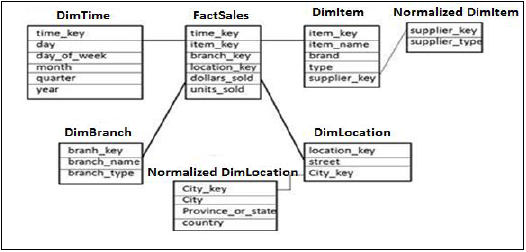# SAP HANA - Schema in Data Warehouse

Schemas are logical description of tables in Data Warehouse. Schemas are created by joining multiple fact and Dimension tables to meet some business logic.

Database uses relational model to store data. However, Data Warehouse use Schemas that join dimensions and fact tables to meet business logic. There are three types of Schemas used in a Data Warehouse −

• Star Schema
• Snowflakes Schema
• Galaxy Schema

## Star Schema

In Star Schema, Each Dimension is joined to one single Fact table. Each Dimension is represented by only one dimension and is not further normalized.

Dimension Table contains set of attribute that are used to analyze the data.

Example − In example given below, we have a Fact table FactSales that has Primary keys for all the Dim tables and measures units_sold and dollars_ sold to do analysis.

We have four Dimension tables − DimTime, DimItem, DimBranch, DimLocationEach Dimension table is connected to Fact table as Fact table has Primary Key for each Dimension Tables that is used to join two tables.

Facts/Measures in Fact Table are used for analysis purpose along with attribute in Dimension tables.

## Snowflakes Schema

In Snowflakes schema, some of Dimension tables are further, normalized and Dim tables are connected to single Fact Table. Normalization is used to organize attributes and tables of database to minimize the data redundancy.

Normalization involves breaking a table into less redundant smaller tables without losing any information and smaller tables are joined to Dimension table.In the above example, DimItem and DimLocation Dimension tables are normalized without losing any information. This is called Snowflakes schema where dimension tables are further normalized to smaller tables.

## Galaxy Schema

In Galaxy Schema, there are multiple Fact tables and Dimension tables. Each Fact table stores primary keys of few Dimension tables and measures/facts to do analysis.In the above example, there are two Fact tables FactSales, FactShipping and multiple Dimension tables joined to Fact tables. Each Fact table contains Primary Key for joined Dim tables and measures/Facts to perform analysis.